# =ARRAYVAL(sample, [options])

Use ARRAYVAL to compute a single value from a solution array obtained by any of the differential equations solvers.

In a dynamical optimization problem, ARRAYVAL can be used to define dynamic constraints on the solution array of the differential equations solver. A constraint formula is typically defined as the difference between the computed value by ARRAYVAL and a target value. The constraint formulas are input to NLSOLVE.

With options, you can apply an element-wise local operation followed by a global operation on the solution values you want to constrain.

### Required Inputs

sample a reference to a single or range of cells within an array result.

If sample contains multiple values, then you must also define at least the second parameter.

### Optional Inputs

goper a reference to a formula to apply to the selected data in sample as a whole such as computing maxima or minima. The formula must reference the data using the variable VAR1 and evaluate to a scalar value.

For example, to compute the maximum of the data, define the formula as =MAX(VAR1). As a convenience, typical global operations such as computing maximum, minimum or mean can be specified by the string MIN, MAX and MEAN without the need to define a formula.

loper a reference to a formula to apply to each element of the data in sample. The formula must reference the data elements using the variable VAR1.

For example, to take the absolute value of the sample elements define the formula =ABS(VAR1).

Consider the array result shown in Table 1 from the simulation of an initial value pendulum problem with IVSOLVE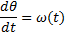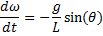With the following parameters: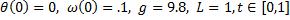A B C 6 T1 theta omega 7 0 0 0.1 8 0.066667 0.006619 0.097835 9 0.133333 0.012949 0.091411 10 0.2 0.018719 0.081034 11 0.266667 0.023675 0.067133 12 0.333333 0.027606 0.050324 13 0.4 0.030336 0.031328 14 0.466667 0.031752 0.010976 15 0.533333 0.031789 -0.00985 16 0.6 0.030448 -0.03025 17 0.666667 0.027784 -0.04934 18 0.733333 0.023917 -0.06629 19 0.8 0.01901 -0.08036 20 0.866667 0.013279 -0.09095 21 0.933333 0.006971 -0.09759 22 1 0.000361 -0.09999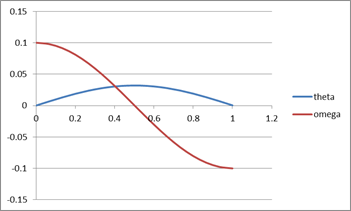You can use ARRAYVAL() to extract or compute values of interest from the simulation result:

 Objective Formula Result Find the maximum value of theta ARRAYVAL(B7:B22,"max")= 0.031789028 Find the absolute minimum of omega =ARRAYVAL(C7:C22,"min",H14) 0.009852877 H14 =ABS(VAR1) Find the mean value of the square of omega =ARRAYVAL(C7:C22,"mean",H18) 0.005295633 H18 >=VAR1^2 Find the total sum of omega =ARRAYVAL(C7:H22) 0.005398066 H22 =SUM(VAR1)

When solving a parametrized dynamical optimization problem, ARRAYVAL is used to define constraints on the dynamical system solution typically as the difference between a computed value by ARRAYVAL and a target value. You can then use the nonlinear solver NLSOLVE to find optimal values for the design parameters that will minimize the differences in your defined constraints.

Question or Comment? Email us:
support @ excel-works.com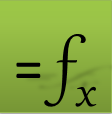ExceLab: Transforming Excel into a Calculus Power House
ExceLab functions and methods are protected by USA Patents 9286286, 9892108, 10114812 and pending.